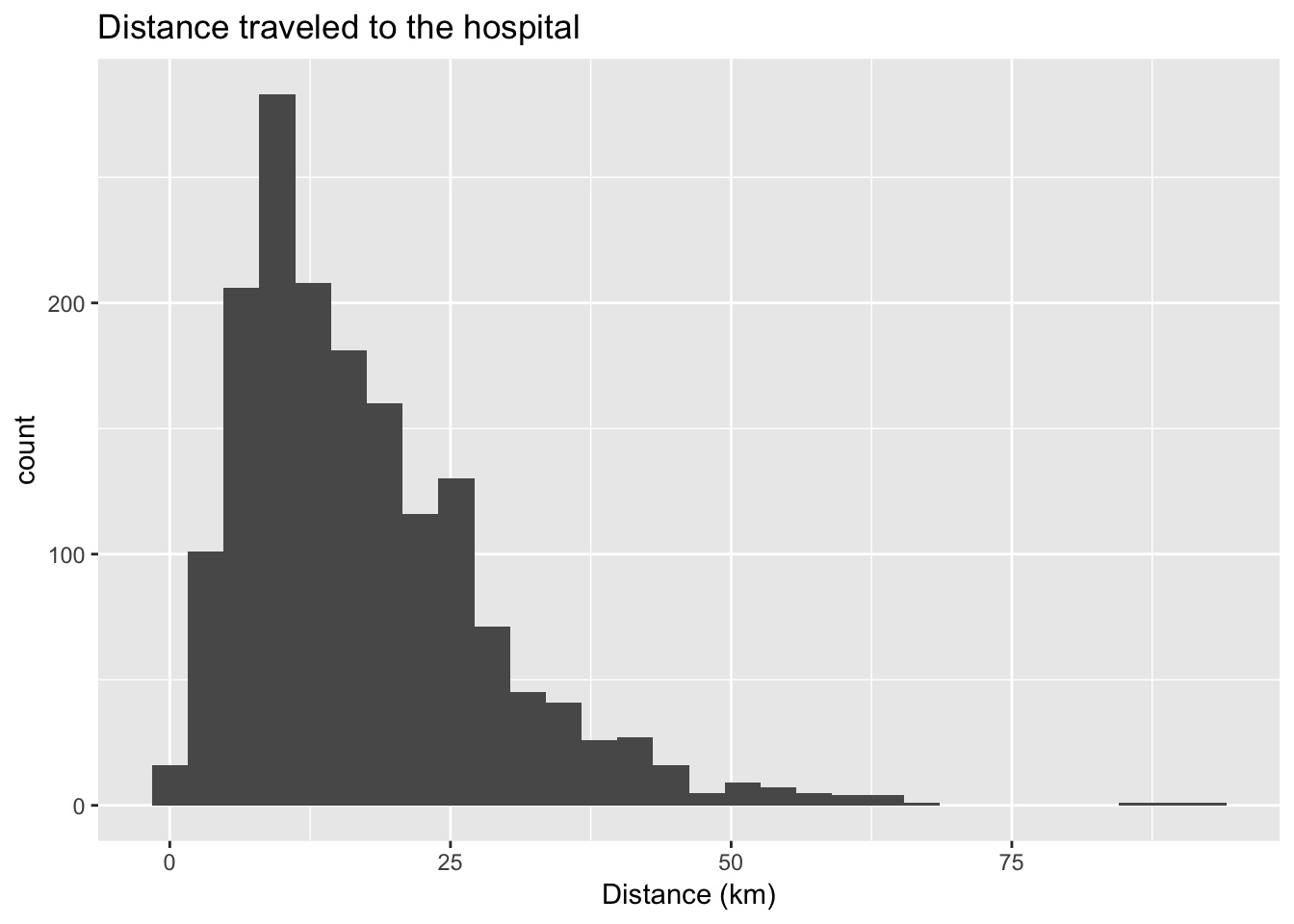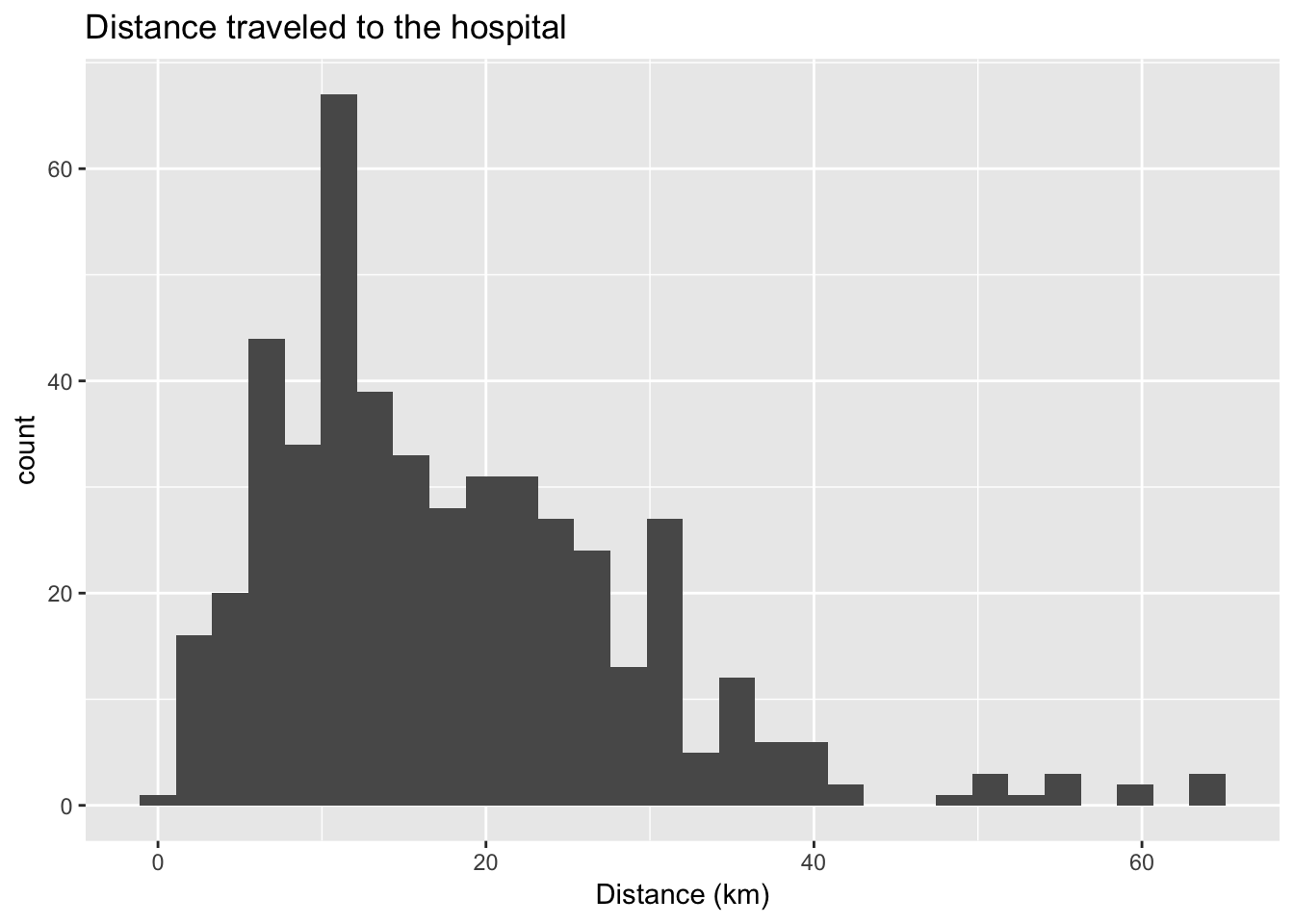# Motivations and goals

We rarely receive data in analysis-ready form with all of the variables we need, the right subset of observations, and the right structure. The dplyr package in R, part of the tidyverse family introduced last week, provides a helpful set of data transformation functions that can get us there.

Today, we’re going to start with learning how to use the function filter() and a suite of “logical operators” (‘and’, ‘or’, ‘not’, etc.) to select the rows we want to use from a dataset. We’ll also practice using ggplot to build on last week’s lesson.

We’ll spend the first 15-20 minutes on this lesson. Then, we’ll shift to discussing coding questions from this week’s lab and problem set.

# Set-up

Before we begin, we need to load the packages we’ll be using for this analysis.

If you haven’t installed the tidyverse package yet, you’ll need to do that before you can load it. You can install the package by typing install.packages("tidyverse") into the console of RStudio and then hitting enter.

library(tidyverse)

I found these data using the new Google Dataset Search tool link. Next, we need to load in our data. We’re going to use a dataset from a study of women using emergency medical services (EMS) for third-trimester pregnancy-related complications in India in 2014.

# if you downloaded it

# if not
dat <- read_csv("https://louisahsmith.github.io/R-office-hours/data/OH-02-data.csv")

# Explore the data (and review ggplot while you’re at it)

Let’s start by just looking at what is in this dataset. The function names() shows us the names of the variables in the dataset.

names(dat)

We’re interested in how far women traveled to the health facility with the EMS. Let’s make a histogram of the distance traveled.

Try making a histogram using the code from last week: ggplot(data = dat, aes(distance_to_hospital)) + geom_histogram(). Why doesn’t this work?

You probably got an error term saying Error: StatBin requires a continuous x variable: the x variable is discrete. Perhaps you want stat="count"?

Since this variable is currently formatted as a character, we need to change it to an integer or numeric value before we can make the histogram.

## Change distance variable to numeric
dat$distance_to_hospital <- as.numeric(dat$distance_to_hospital)

## Make histogram
ggplot(data = dat, aes(distance_to_hospital)) +
geom_histogram() +
ggtitle("Distance traveled to the hospital") +
xlab("Distance (km)")How would you describe this distribution?

We can also calculate some descriptive statistics. Note that we tell R to ignore the missing values by typing na.rm=T.

## Mean distance
mean(dat$distance_to_hospital, na.rm=T) ## Sample variance var(dat$distance_to_hospital, na.rm=T)

## Range of distances
range(dat$distance_to_hospital, na.rm=T) # Filter with Boolean operators Let’s say we want to know the distribution of distances traveled by different subsets of women in the dataset. The function filter() allows us to filter observations based on their values. The first argument of filter() is the name of the dataset. The next arguments refer to different restrictions we would like to place on the subset of observations. For example, if we’re only interested in observations from Andhra Pradesh, we can filter the dataset as follows: filter(dat, state == "Andhra Pradesh") If we want to save this subset as it’s own data frame, we can use the code: dat_ap <- filter(dat, state == "Andhra Pradesh") Now, we can re-make the histogram or calculate summary statistics using only data from Andhra Pradesh. ggplot(data = dat_ap, aes(distance_to_hospital)) + geom_histogram() + ggtitle("Distance traveled to the hospital") + xlab("Distance (km)")mean(dat_ap$distance_to_hospital, na.rm=T)
##  18.01044
nrow(dat_ap) # tells us how many rows the new dataset has
##  480

We can identify the observations we’re interested in using comparison operators: >, >=, <, <=, !=, and ==. Note that we use == and not = to denote “equal.” A single equal sign (=) can be used instead of <- to assign values to things in R.

If we only want to analyze data for women who traveled more than 20km, we can use the code filter(dat, distance_to_hospital > 20).

We can also combine multiple conditions using Boolean operators: & (“and”), | (“or”), and ! (“not”).

Let’s say we’re interested in women in Andhra Pradesh and Gujarat who have had previous C-sections. We can identify this group using the code filter(dat, (state=="Andhra Pradesh" | state=="Gujarat") & Prior_csection=="Yes").

We can also write this as: filter(dat, (state %in% c("Andhra Pradesh", "Gujarat")) & Prior_csection=="Yes"). The part that says state %in% c("Andhra Pradesh", "Gujarat") is telling us that we want a state that is part of the list of Andhra Pradesh and Gujarat.

One way to check if you are subsetting the data correctly is to use the table() function. For example table(dat\$state) will tell you the number of observations from each state in your dataset. What would the code be if we were interested in subsetting the data to women with either anemia or hypertension who not in Assam?

# Missing values

In the code above, we ignored missing values when we calculated the mean by using na.rm=T (which you can read as “remove NAs is true.”) If we had tried to calculate the mean without specifying this, R would have told us that the mean itself was NA. This is because, in R, whenever there is a missing value the result of an operation will also be missing.

Let’s say we want to include missing values in our subset. If we’re interested in women who had a previous C-section or are missing, we can subset our data as filter(dat, Prior_csection=="No" | is.na(Prior_csection)).

Note that we don’t say Prior_csection==NA, but this is essentially what is.na() means.

# One last tricky thing

It’s important to be careful when using the Boolean operator ==. The computer can’t store infinite digits, so we find that things we know to be true are not actually true in R!

sqrt(2) ^ 2 == 2
##  FALSE
1 / 49 * 49 == 1
##  FALSE

To get around this, we can use the function near().

near(sqrt(2) ^ 2,  2)
##  TRUE
near(1 / 49 * 49, 1)
##  TRUE

# Exercises

For more exercises like this, refer to Chapter 5 of “R for Data Science” link Here are some exercises to practice using filter() and the Boolean operators.

1. How many women delivered in Gujarat, Assam, or Karnataka and were in the 18-35 age range?

2. Use filter to create separate datasets for women who used EMS services in urban, rural, and tribal areas. Calculate the mean distance traveled in each of these groups.

3. An convenient way to compare distributions of a variable for different subsets in your data is to use to use ggplot with facet_wrap. Make a histogram of the distance traveled to the facility (using the full dataset) and then add facet_wrap(~incidence_area) to see how this works.